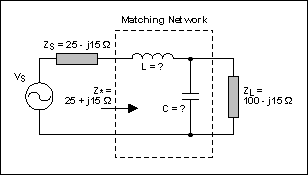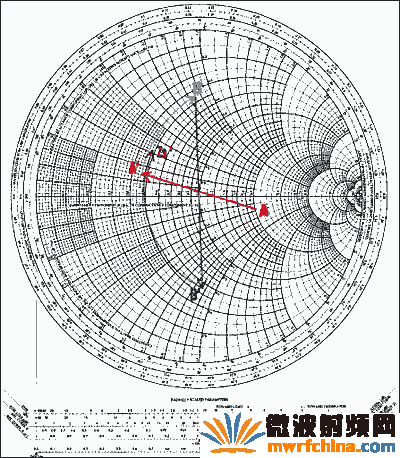## 阻抗匹配与史密斯(Smith)圆图：基本原理

2022-07-08 18:08发布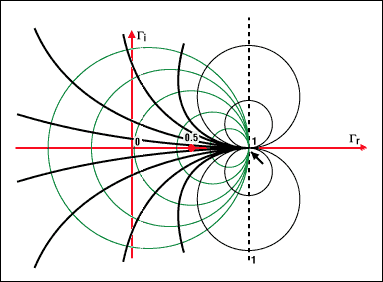## 基础知识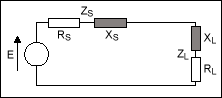## 史密斯圆图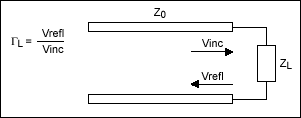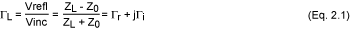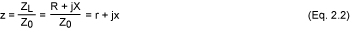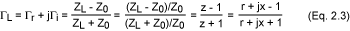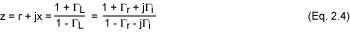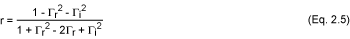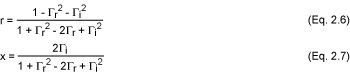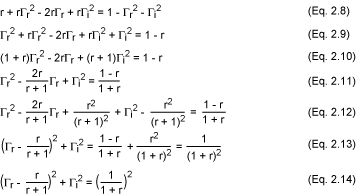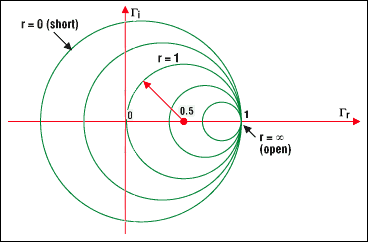• 所有的圆周只有一个相同的，唯一的交点(1, 0)

• 代表0也就是没有电阻(r = 0)的圆是最大的圆

• 无限大的电阻对应的圆退化为一个点(1, 0)

• 实际中没有负的电阻，如果出现负阻值，有可能产生振荡

• 选择一个对应于新电阻值的圆周就等于选择了一个新的电阻

## 作图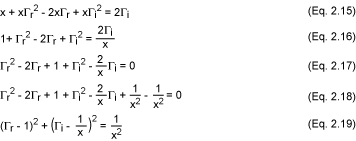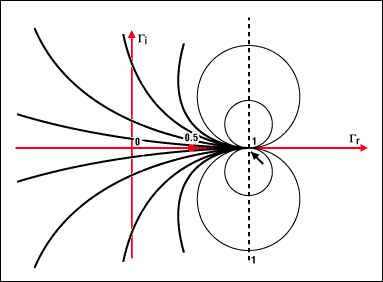## 推论

 Z1 = 100 + j50 Z2 = 75 - j100 Z3 = j200 Z4 = 150 Z5 = (开路) Z6 = 0 (短路) Z7 = 50 Z8 = 184 - j900

 z1 = 2 + j z2 = 1.5 - j2 z3 = j4 z4 = 3 z5 = 8 z6 = 0 z7 = 1 z8 = 3.68 - j18

 1 = 0.4 + 0.2j 2 = 0.51 - 0.4j 3 = 0.875 + 0.48j 4 = 0.5 5 = 1 6 = -1 7 = 0 8 = 0.96 - 0.1j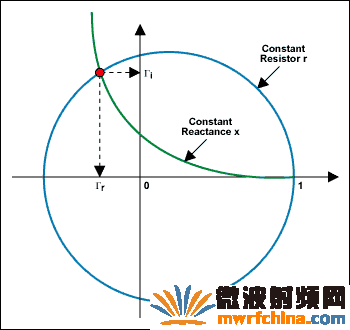## 用导纳表示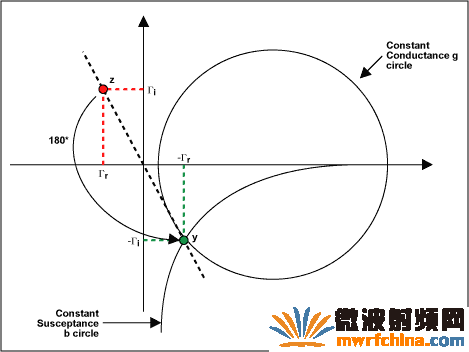## 导纳圆图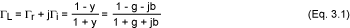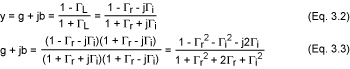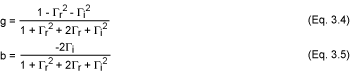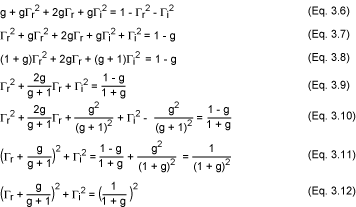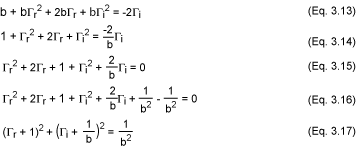## 求解等效阻抗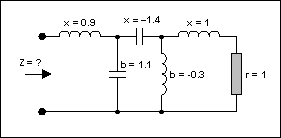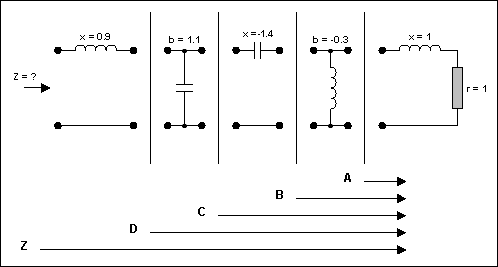## 逐步进行阻抗匹配